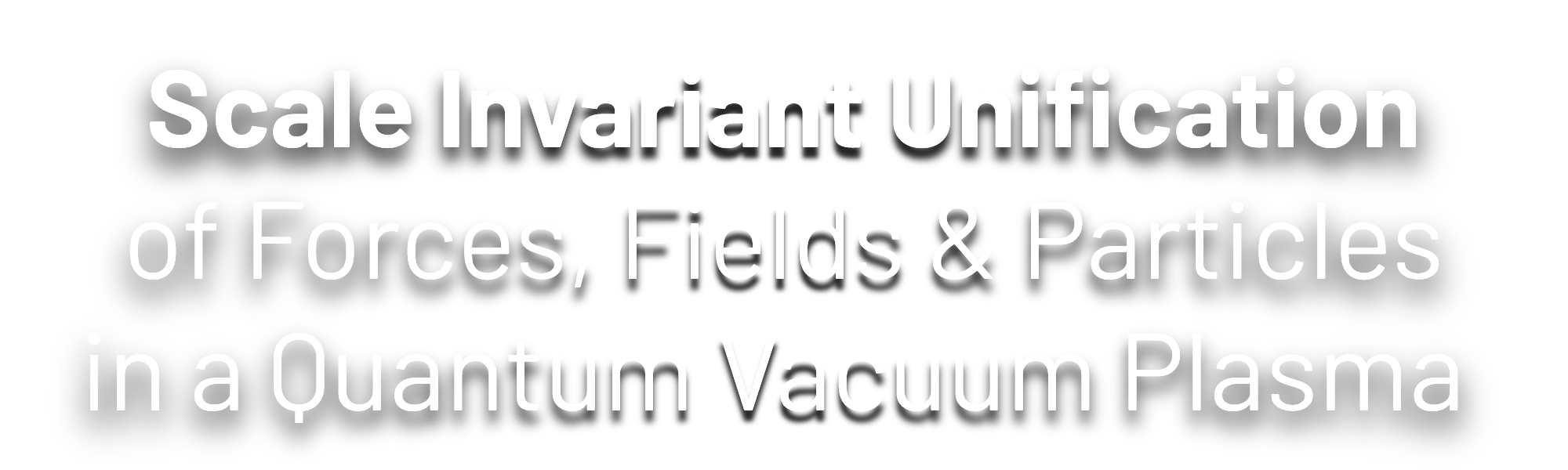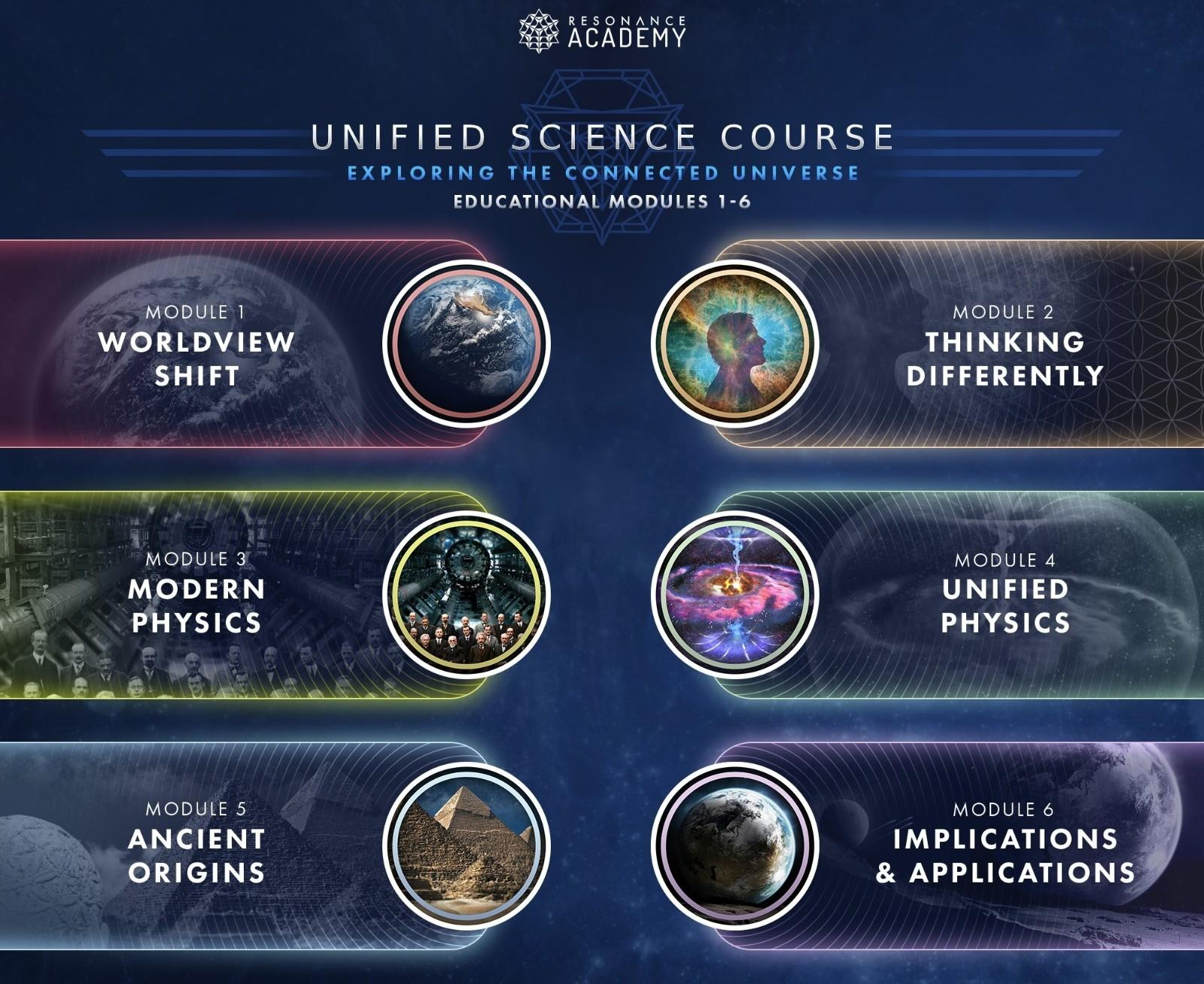#### NEW RESEARCH PAPER## Abstract

View Pre-Print Abstract

From Bekenstein(1)-Hawking(2), Susskind(3) and 't Hooft(4) development of the holographic principle, we consider a statistical entropy and thermodynamics approach of a surface-to-volume generalized holographic ratio defined in previous work (5)(6)(7). Applying this analysis, we chart a first order approximation of a scaling coefficient utilizing a face-centered cubic crystalline structure representation of spacetime. The resulting scaling from the Planck scale to the universal scale finds a surprisingly periodic fit to organize matter in the universe. As a result, we can compute exact values defining the fundamental scaling factors of physical interactions. By applying them to radii and masses at the hadronic, electronic and Hubble Constant Ho scale we find results consistent with current measurements.

Utilizing these scaling factors, we compute a value for the gravitational constant G with an accuracy of ten significant figures 10-10, representing a first analytical solution for G , which is typically only known to five significant figures of accuracy from experiment. The accuracy of the value can be confirmed by computing the Rydberg constant, known from measurements to 10-12 accuracy, utilizing our scaling factor and our derived value of G . As a result, we are now able to derive all Planck units to 10-10 significant figures.

The relationship between the constants through our computed scaling factors derived from the fundamental holographic ratio Φ is further confirmed by the precise computation of the mass of the electron, the radius of the electron, the Bohr magnetron and the mass of the proton, all known to 10-10 by measurements. As a result, we can demonstrate a clear relationship between the classical scale of the gravitational constant and the quantum energy spectra of the atomic scale from first principle of theoretical tenets alone.

As a result of our scaling law, we now have a geometric interpretation of the fine structure constant and the proton to the electron mass ratio and find the Koide formula to be in a direct relationship to the scaling factor. Therefore, utilizing this formula we compute an exact solution for α and μ. We then validate this value to 10-13 accuracy by predicting the electron g-factor ge . As a result, we are as well able to predict the vacuum permittivity constant ε0 as an exact value which is known to 10-10 accuracy.

Furthermore, considering the 2019 redefinition of the SI base units and the natural Planck units we are now able to unify all the measurements into one analytical framework. Thus, by having only one measurement, such as the Rydberg constant, one can compute all the other values to 10-12 accuracy.

This opens the way to the unification of forces, which we scale and find a direct correlation between the forces coupling constants and our holographic ratio Φ at all scales. Resulting in the unification of particles, fundamental constants and forces in a unified theoretical framework based on a fractalization of spacetime defined by an entropic principle related to the surface-to-volume holographic ratio Φ.

References

1. J. D. Bekenstein, Phys. Rev. D 23, 287 (1981).

2. S. W. Hawking, Nature 248, 30 (1974). S. W. Hawking, Commun. Math. Phys. 43, 199 (1975).

3. L. Susskind, J. Math. Phys. 36, 6377 (1995)

4. G. ’t Hooft, “Dimensional reduction in quantum gravity,” arXiv:gr-qc/ 9310026 (1993). G. ’t Hooft, Basics and Highlights in Fundamental Physics (Proceedings of the International School of Subnuclear Physics, Erice, Sicily, Italy, 2000).

5. Quantum Gravity and the Holographic Mass, Haramein, N. (2012). Quantum Gravity and the Holographic Mass, Physical Review & Research International, ISSN: 2231-1815, Page 270-292

6. The Electron and the Holographic Mass Solution, Val baker, A.K.F, Haramein, N. and Alirol, O. (2019). The Electron and the Holographic Mass Solution, Physics Essays, Vol 32, Pages 255-262.

7. Resolving the Vacuum Catastrophe: A Generalized Holographic Approach, Haramein, N & Val Baker, A. K. F. (2019). Resolving the Vacuum Catastrophe: A Generalized Holographic Approach, Journal of High Energy Physics, Gravitation and Cosmology, Vol.05 No.02(2019), Article ID:91083, 13 pages

## Featured Media